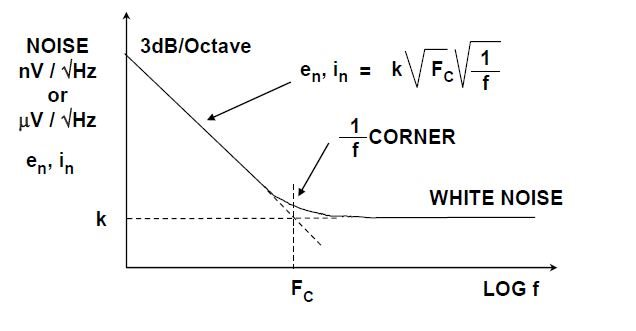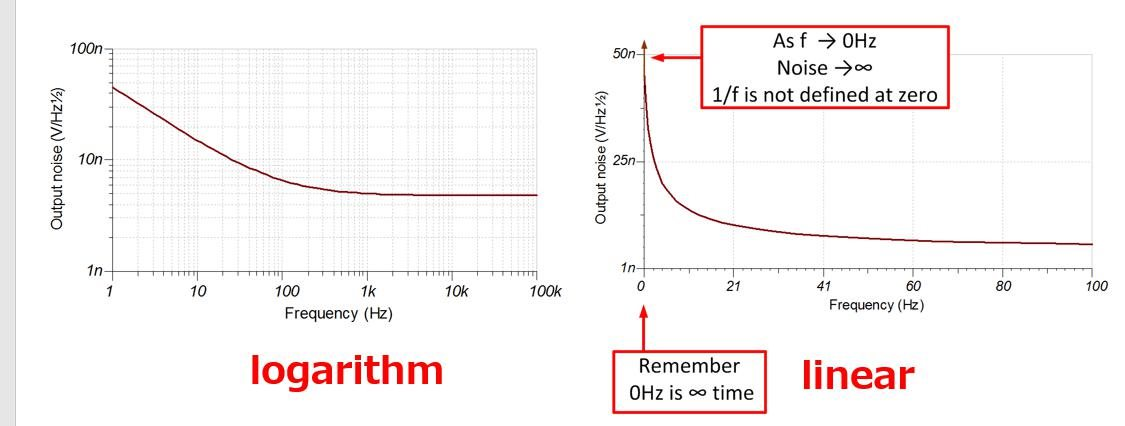If you have a related question, please click the "Ask a related question" button in the top right corner. The newly created question will be automatically linked to this question.

• TI Thinks Resolved

# REF5025: 1/f noise

Part Number: REF5025

Hi,

REF5025 outputs DC signla(=0Hz).

However, 1/f noise is infinity at 0Hz.

How should we consider about 1/f noise of reference voltage?

Best Regards,

Kuramochi

• Hi Kuramochi

If you see noise density plot of any semiconductor device, it will look as follow -As graph shows , low frequency noise is always proportional to 1/f . From the graph we can conclude that value of low frequency noise depends on FC and K.

where k = white noise (v/rt hz)

FC is cutoff frequency for flicker noise

The rms noise can be calculated by area measurement by taking care of Y axis unit (V/r hz ).

So 1/f noise means the slope is inversely proportional to frequency but it doesn't go to infinite. Its final value depends on white noise and flicker noise cutoff frequency.

Regards

Trailokya

• In reply to Trailokya Rai:

Trailokya-san,

>The rms noise can be calculated by area measurement by taking care of Y axis unit (V/r hz ).

This is logarithm scale, right?

I think that it cannot be calculate because start point of x axis is not 1Hz but 0Hz.> Its final value depends on white noise and flicker noise cutoff frequency.

What does "cutoff frequency" mean?

Best Regards,

Kuramochi

Hi Kuramochi San

FC is the frequency at which the noise start rising as you can see in the earlier diagram.It is a figure of merit.

In logarithmic scale . start point can be some 10^-N {where N is an integer} but it is not defined at 0.

RMS noise contribution for <0.1Hz frequency noise is not significant because X axis is very small.

So the flicker noise is calculated from 0.1Hz to 10Hz usually.

Regards

Trailokya

• In reply to Trailokya Rai:

Hello Trailokya-san,

>because X axis is very small.

I can not understand it. Could you please explain in detail?

Best Regards,

Kuramochi